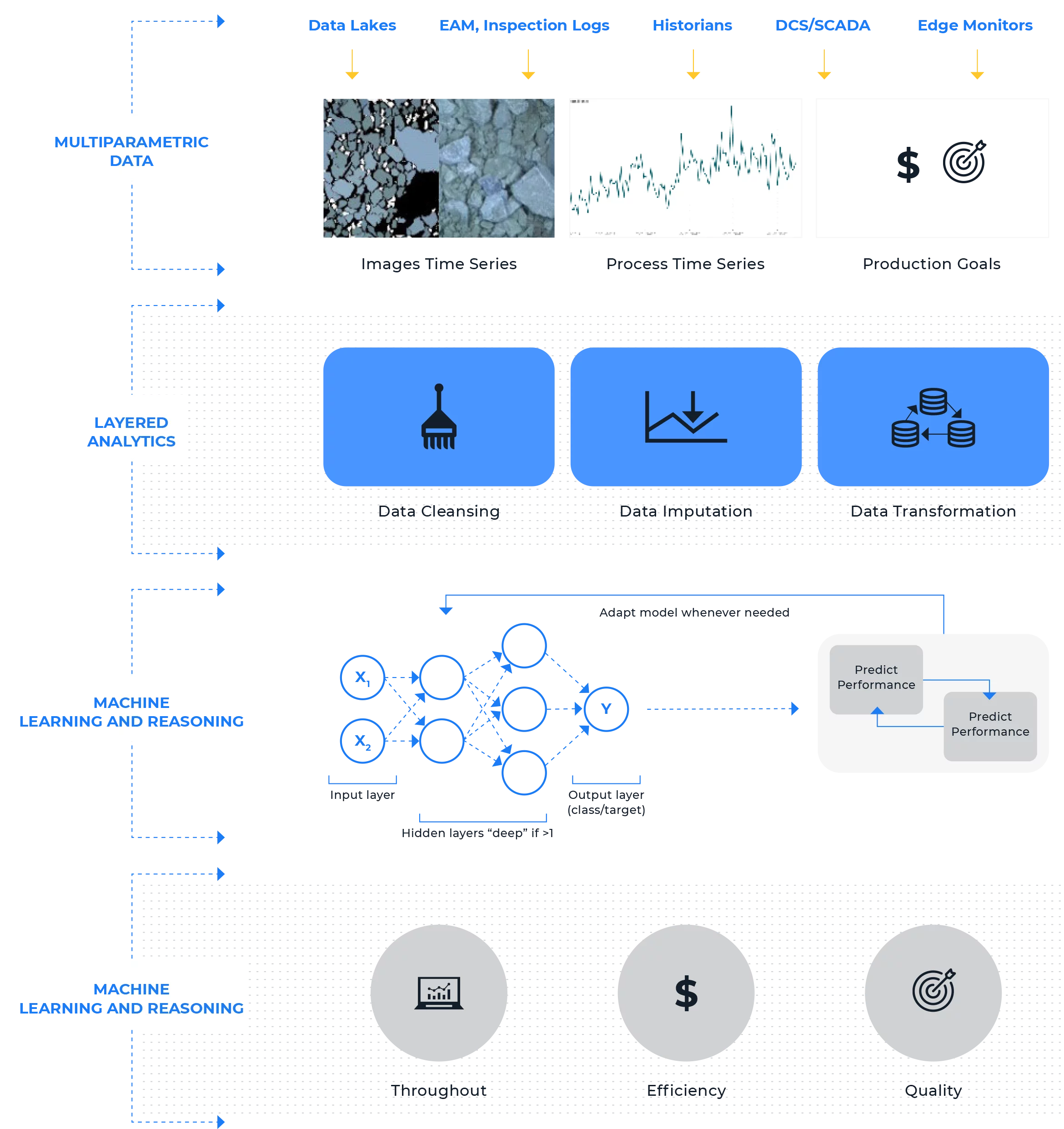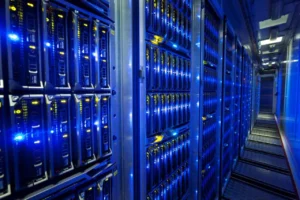# Artificial Intelligence Primer

Blog | 29 Apr 2020

### What is “Artificial Intelligence”?

Artificial Intelligence (AI) is an area of computer science that emphasizes the creation of intelligent machines that work and react like humans. Researchers design computers with artificial intelligence to perform activities such as:

• Speech recognition
• Planning
• Learning
• Problem solving

Knowledge engineering is a core part of AI research. Machines can often act and react like humans only if they have sufficient information relating to the world. Artificial intelligence must have access to objects, categories, properties, and relations between all of them to implement knowledge engineering.

### What is “Machine Learning”?

Machine learning is also a core part of AI. Learning without any supervision requires an ability to identify patterns in streams of inputs, whereas learning with adequate supervision involves classification and numerical regressions. Classification determines the category an object belongs to, and regression deals with obtaining a set of numerical input or output examples, thereby discovering functions enabling the generation of suitable outputs from respective inputs. Mathematical analysis of machine learning algorithms and their performance is a well-defined branch of theoretical computer science often referred to as computational learning theory.

Deep Learning is a subset of Machine Learning that has networks capable of learning unsupervised from data that is unstructured or unlabeled. Deep Learning algorithms attempt to draw similar conclusions as humans would by continually analyzing data with a given logical structure. In performing this analysis, deep learning uses a multi-layered structure of algorithms called neural networks. Artificial neural networks have unique capabilities that enable deep learning models to solve tasks that machine learning models can never solve.

### What is the difference between “Deep Learning” and “Machine Learning”?

Flat algorithms are the basis for traditional Machine Learning methods meaning that they cannot be applied directly to raw data. Instead, they require a preprocessing step called feature extraction. Feature extraction is usually quite complex and requires detailed knowledge of the problem domain. The preprocessing step must be adapted, tested, and refined over several iterations to generate optimal results. In comparison, Deep Learning does not require the feature extraction step. The layers can learn an implicit representation of raw data directly and on their own. During the training process, the neural network obtains the best possible abstract representation of the input data. In other words, Deep Learning models require little to no manual effort to perform and optimize the feature extraction process. Massive amounts of data (i.e., big data) power Deep Learning and it’s models increase their accuracy as the amount of training data increases.

### What is Python?

It is an interpreted, high-level, general-purpose programming language. Python’s design philosophy emphasizes code readability (meaning that it looks like English sentences). Its language constructs and object-oriented approach aim to help programmers write clear, logical code for small and large-scale projects. Python supports multiple programming methods, including procedural, object-oriented and functional programming. Many describe it as a “batteries included” language due to its comprehensive standard library.

### How does Performance 360 use AI?

Performance 360 uses a carefully curated library of methods leveraging best-in-class open source frameworks such as Tensorflow, Keras, SciPy to support deep learning    algorithms, otherwise known as deep artificial neural networks (a subset of artificial intelligence). What makes them “deep”? These networks train with data and self-learn – without needing human programming. Further, our Machine Learning approach employs:

• Convolutional neural networks (CNN) that help analyze and classify imagery,
• Long short-term memory (LSTM) networks for classifying, processing, and making predictions based on time-series data.
• Autoencoders blended with K-Nearest Neighbor (KNN), Support Vector Regression (SVR), and Arima computational techniques.### Artificial neural networks (ANN)

Computational algorithms intended to simulate the behavior of biological systems compose of “neurons.” It is capable of machine learning as well as pattern recognition. The neural network is an oriented graph consisting of nodes. It is an information processing technique that includes a large number of connected processing units that work together to process information.

### Adaptive artificial neural networks (AANN)

When an artificial neural network learns, the weights between the neurons (or nodes) are changing, and so do the connection strengths. The typical neural network architecture consists of several layers. The first layer is the input layer. The last layer is the output layer (meaning the result that the neural network came up with). To obtain a prediction, the neural must perform certain mathematical operations in the layers between the input and output. These layers are known as the “hidden layers.”

Once the network has its prediction, it goes through another step of comparing this prediction to the actual ground truth. The difference between the two is called the loss function. Minimizing the loss function directly and automatically leads to more accurate predictions of the neural network. In other words, the network keeps improving its ability to predict as it processes more data (otherwise known as “self-learning” or “unsupervised learning.”

### A Convolutional Neural Network (CNN)

is a class of deep learning networks that developers use to analyze visual imagery and are frequently working behind the scenes in image classification. Image classification is the process of taking an input (such as a picture) and outputting a class or a probability that the input is a particular class. A CNN convolves learned features with input data, and it requires very little preprocessing. They can learn the filters that have to be hand-made in other algorithms. A CNN extracts features from images and learns by training on a set of images. Combined with an artificial neural network, CNN and ANN techniques combine image features with more attributes resulting in a highly accurate class prediction.

### Long short-term memory (LSTM) is an artificial recurrent neural network (RNN) architecture

used in the field of deep learning. LSTM networks are well-suited to classifying, processing, and making predictions based on time- series data. Because there can be lags of unknown duration between important events in time series, exploding and vanishing gradient problems can arise when training tradition RNNS. LSTMs are designed to handle this phenomenon. RNN builds models using a recursion technique. Because of their internal memory, RNNs can remember important things about the input they received. This feature allows them to be very precise in predicting what’s coming next. Lastly, recurrent neural networks can form a much deeper understanding of a sequence and its context compared to other AI algorithms.

### Support vector regression (SVR)is rooted in statistical learning. As in classification, support vector regression (SVR) is characterized by the use of kernels, sparse solution, and VC control of the margin and the number of support vectors. Although less popular than SVM, SVR has been proven to be a useful tool in real-value function estimation. As a supervised-learning approach, SVR trains using symmetrical loss function, which equally penalizes high and low misestimates.

### The Arima computational technique

is a popular and widely used statistical method for time-series forecasting. Arima is an acronym that stands for AutoRegressive Integrated Moving Average. It is a class of model that captures a suite of different standard temporal structures in time-series data.

### How does APM 360 use AI?

Similarly, APM 360™ uses  a carefully curated library of methods leveraging best-in-class open source frameworks such as TensorFlow, Keras, SciPy and Symphony Ayasdi’s Topological Data Analysis (TDA). Enabled with fit-for-purpose supervised and unsupervised AI models that harness data from high-frequency vibrations to process, APM 360™ analytics provide accurate predictions of asset performance. Our anomaly detection is coupled with a fault (FMEA( library) for automated cause analysis and advisories. Examples of AI methods employed are:

• Unsupervised Learning methods
• Autoencoders blended with Topological Data Analysis (TDA)
• Principal component analysis (PCA) to identify a smaller number of uncorrelated variables from a more extensive data set.
• Supervised learning methods
• Recurrent neural networks with KNN
• Principal component analysis (PCA) to identify a smaller number of uncorrelated variables from a more extensive data set.

### Principal component analysis (PCA)

identifies a smaller number of uncorrelated variables known as principal components from a more extensive data set. Developers widely use this technique to emphasize variation and capture strong patterns in a data set. Data scientists use principal component analysis as a tool in predictive models and exploratory data analysis.

### One class support vector machine (OCSVM)

is particularly useful in scenarios where there is a lot of “normal” data and not many cases of the anomalies that need detecting. For example, if a bank needed to detect fraudulent transactions, it may not have many instances of fraud that it could use to train a typical classification model; it might have many cases of good transactions.

Data scientists us the One-Class Support Vector Model module to create the model and then train the model. The dataset they use for training can contain all or mostly normal cases. They can then apply different metrics to identify potential anomalies. For example, they might use a large dataset of good transactions to identify cases that possibly represent fraudulent transactions.

### Physics-based modeling

or simulation tools allow developers to not only predict what will happen but why it will happen, unlike building an actual prototype. This approach offers the insights developers need to make the correct choices. In other words, simulation can help them choose between good ideas and bad ones. Implementing a digital twin for an asset requires a solution, to begin with, the asset’s physics-based model. This approach allows the twin to simulate the product’s field performance in real-time using the same sensor information that the physical asset is experiencing. This real-time simulation with real-world data can enable real-time analytics, delivering better business outcomes through efficiency gains and reduction in unplanned downtime.

### Support vector machines (SVMs)are supervised learning models that analyze data and recognize patterns. Data scientists use them for both classification and regression tasks. Typically, the data scientist gives the SVM algorithm is a set of training examples labeled as belonging to one of two classes. The basis of the SVM model is to divide the training sample points into separate categories by as wide a gap as possible while penalizing training samples that fall on the wrong side of the difference. The SVM model then makes predictions by assigning points to one side of the difference or the other.

Sometimes data scientists use oversampling to replicate the existing samples so they can create a two-class model. But, it is impossible to predict all the new patterns of fraud or system faults from limited examples. Therefore, in one-class SVM, the support vector model is trained on data that has only one class, which is the “normal” class. It infers the properties of normal cases and from these properties can predict which examples are unlike the normal examples. This technique is useful for anomaly detection because the scarcity of training examples is what defines anomalies: that is, typically, there are very few examples of the network intrusion, fraud, or other anomalous behavior.